Lattice enthalpy

• In AS we looked at ionic bonding and learned that:

Ionic bonds are strong

Due to strong electrostatic forces of attraction

• This means that the melting and boiling points of ionic compounds are very high.

• This is due to the lattice enthalpy, DH qLE:

Definition – Lattice enthalpy

The lattice enthalpy is the enthalpy change when 1 mole of an ionic compound is formed from its gaseous ions under standard conditions (298K, 100kPa).• For sodium chloride:

 Na+(g) + Cl-(g) g NaCl(s) DHqLE = -781 KjMol-1

Features of lattice enthalpy: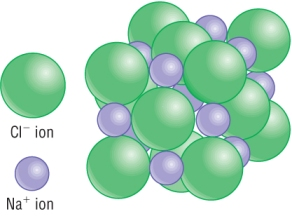Lattice enthalpy is an exothermic process as large amounts of energy is released upon the formation of the lattice from gaseous ions. Lattice enthalpy indicates the strength of ionic bonds in a compound lattice structure. Lattice enthalpy is impossible to measure directly as it is impossible to have gaseous ions. Covalent compounds cannot have lattice enthalpies due to the nature of the bonding.

• As it is not possible to calculate directly, a special type of Hess's cycle is used.

• This is called a Born - Haber cycle.

• These cycles require many more types of enthalpy changes:

Key enthalpy changes:

1)  Standard enthalpy change of formation, DH qf :1 mole of compound is formed from its constituent elements in their standard state.

Example - usually exothermic

K(s)     +    1/2Cl2(g)   à        KCl(s)            DH qf  =  - 437 KJ Mol-1

2)  The standard enthalpy change of atomisation,DH qat :1 mole of gaseous atoms are formed from the element in its standard state.

Example - Always endothermic as bonds have to be broken:

Mg(s)  à  Mg(g)            DH qat  =  +148 KJ Mol-1

3)  First ionisation energy, DH qI1 :1 mole of gaseous 1+ ions is formed from gaseous atoms

Example - Always endothermic as an electron has to overcome the attraction from the nucleus:

Li(g)    à  Li+(g)    +  e-            DH qI1  =  + 520 KJ Mol-1

4)  Second ionisation energy, DH qI2 :1 mole of gaseous 2+ ions is formed from 1 mole gaseous 1+ ions

Example - Always endothermic as an electron has to overcome the attraction from the nucleus:

Ca1+(g)    à  Ca2+(g)    +  e-            DH qI2  =  + 1145 KJ Mol-1

5)  First Electron affinity, DH qEA1 :1 mole of gaseous 1- ions formed from gaseous atoms

Example - Is exothermic as an electron is attracted to the outer shell by the nucleus:

Cl(g)  +  e-  à  Cl-(g)              DH qEA1  =  - 349 KJ Mol-1

6)  Second Electron affinity, DH qEA2 :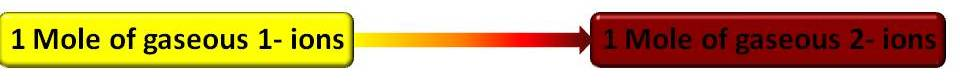1 mole of gaseous 2- ions formed from 1 mole gaseous 1- ions

Example - Is endothermic as the electron being put in is repelled by the 1- ion:

O-(g)  +  e-  à  O2-(g)              DH qEA2  =  - 798 KJ Mol-1

Qu 1 - 3  P 167

Constructing Born - Haber cycles

• Born - Haber cycles are basically a form of Hess's cycle particularly for calculating lattice enthalpies.

• Start with the elements at 'zero energy' - called the datum line.

• Endothermic processes go up

• Exothermic processes go down

• A simple view of the Born - Haber cycle is shown below:• Route 2 is actually a multi - step process changing elements to gases then to ions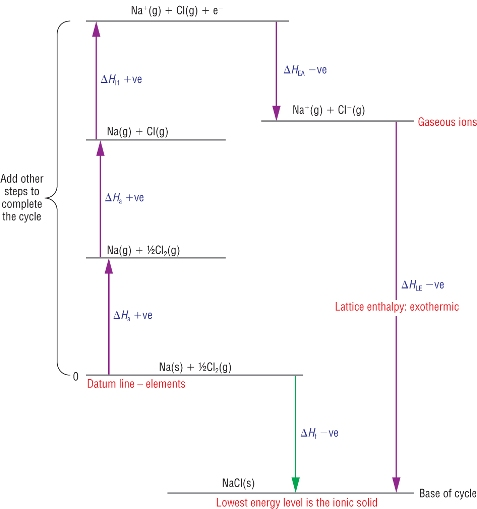• Route 2 has to be calculated in stages using a combination of enthalpy changes.

• Taking one element at a time use enthalpy changes to convert each element into gaseous atoms.

• From there, use successive ionisation energies or electron affinities to convert the gaseous atoms into gaseous ions.

• Apply Hess’s cycle to calculate the Lattice energy.

Calculation:

Route 1     =     - Route 2  +  Route 3

Route 1     =     Route 3  -  Route 2

Lattice enthalpy, DHqLE         =         DHqf      -         Route 2 (the rest)

Or better - rearranged:

 DHqLE = DHqf - (SUM OF DHq THE REST)

Other rules:

2 ions of the same formula, MgCl2You need to count the enthalpies from the atom in its standard state to the ion in its gaseous state TWICE.

More than 1 charge on the ion – you need to 2 ionisation energies or electron affinities:

eg  Mg  à  Mg+  à  Mg2+                      or                            O  à  O-  à  O2-

Qu 1 - 3  P169

Born - Haber cycle calculation

• Worked examples:

1)  Caesium chloride: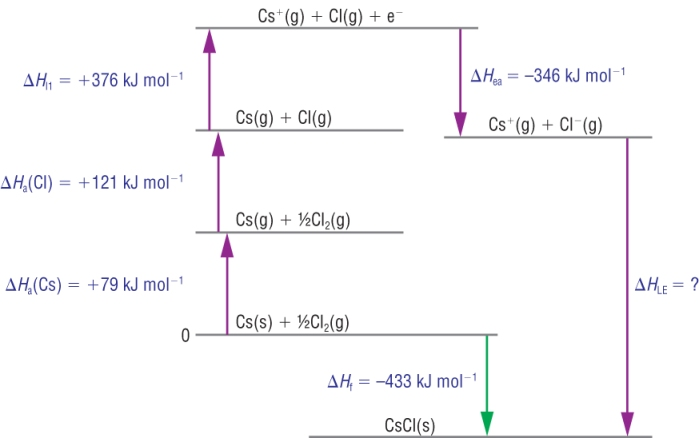Calculation:

 DHqLE = DHqf - (SUM OF DHq THE REST) DHqLE = - 433 - ( +79 + 121 + 376 +  - 346) DHqLE = - 433 - ( +79 + 121 + 376 - 346) DHqLE = - 663 Kj mol-1

2)  Sodium oxide: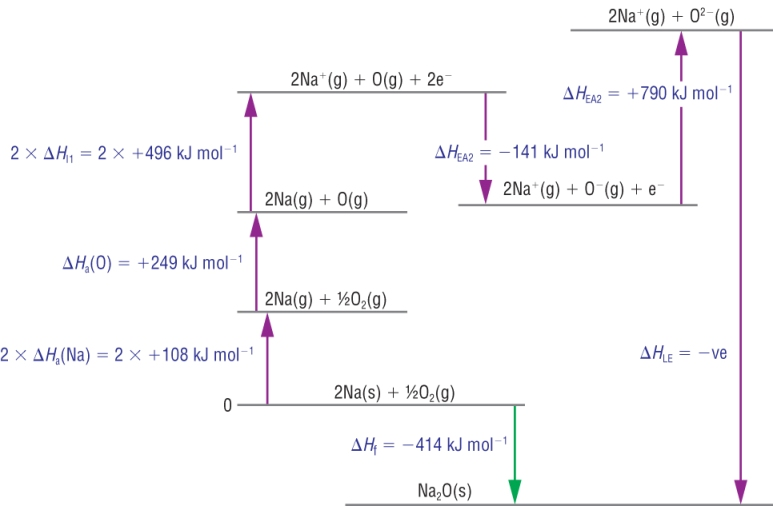Calculation:

 DHqLE = DHqf - (SUM OF DHq THE REST) DHqLE = - 414 - ( +216 + 249 + 992 + - 141 + 790) DHqLE = - 414 - ( +216 + 249 + 992 - 141 + 790) DHqLE = - 2520 Kj mol-1

Qu 1  P 171

3)  Calcium chloride:

• These cycles can be used to calculate other unknowns: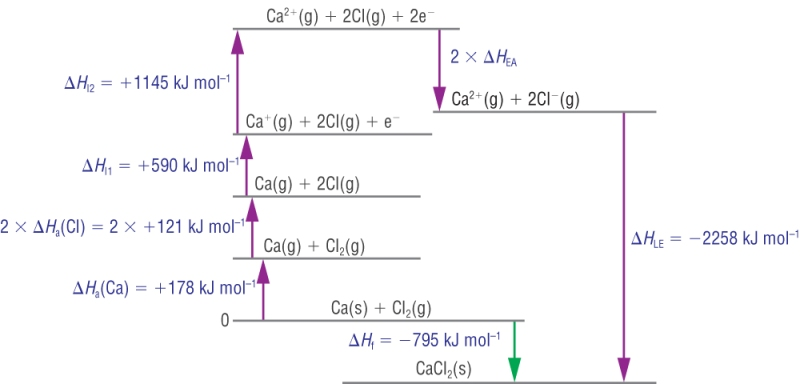Calculation:

 DHqLE = DHqf - (SUM OF DHq THE REST) -2258 = - 795 - ( +178 + 242 + 590 + 1145 + (2 x DHqEA)) -2258 = - 795 - (+ 2155 + (2 x DHqEA)) -2258 = - 795 - 2155 - (2 x DHqEA) -2258 = - 2950 - (2 x DHqEA) 2 x DHqEA = - 2950 +  2258 2 x DHqEA = - 692 DHqEA = - 346 Kj mol-1

4)  Copper (II) oxide: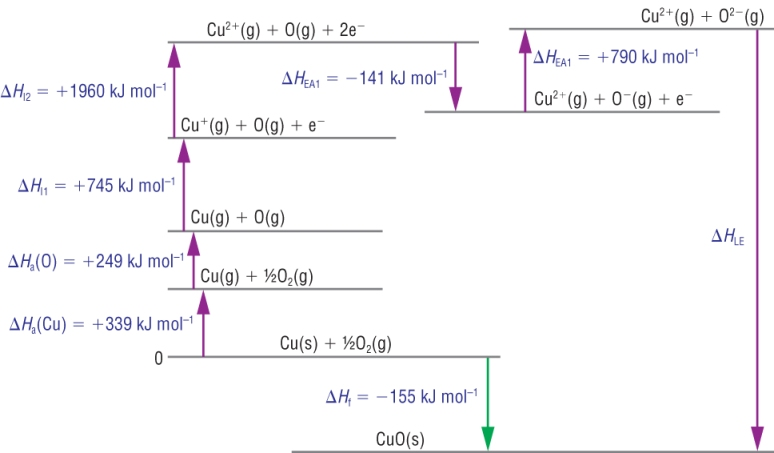Calculation:

 DHqLE = DHqf - (SUM OF DHq THE REST) DHqLE = - 155 - ( +339 + 249 + 745 + 1960 + -141 + 790) DHqLE = - 155 - ( +339 + 249 + 745 + 1960 - 141 + 790) DHqLE = - 4097 Kj mol-1

Qu 1  P173

• When ionic compounds dissolve in water the ions separate.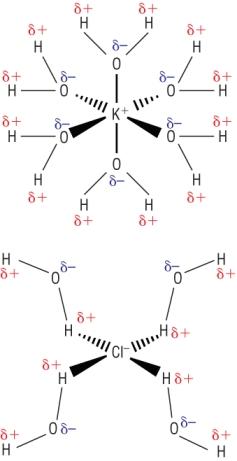If the electrostatic forces of attraction are so strong, how is this possible? The clue comes with the temperature changes that occur when they dissolve. Calcium chloride gives us an exothermic reaction. Ammonium nitrate gives us an endothermic reaction. The energy produced when water surrounds the ions must be about the same as the electrostatic forces of attraction between the ions. If there is energy left over it is given out as heat energy. If there is not enough energy it is taken in from the surroundings These can all be calculated in another type of Hess's cycle but some other enthalpy changes are required first:

1)  Enthalpy change of solution, DHqs1 mole of a compound is dissolved in water under standard conditions

Example - This process can be endothermic or exothermic:

KCl(s)  +  aq   à  K+(aq)   +      Cl-(aq)

2)  Enthalpy change of hydration, DHqhyd1 mole of aqueous ions are formed from their gaseous ions under standard conditions

Example - This process is an exothermic process:

K+(g)  +  aq   à  K+(aq)

Cl-(g)  +  aq   à  Cl-(aq)

What happens when a solid dissolves:

• In terms of a Hess's cycle:

1)  Ionic lattice breaks down into gaseous ions, Ein:

• This is the opposite of the lattice enthalpy:

 K+(g) + Cl-(g) g KCl(s) DHqLE = - 771 KjMol-1
• Opposite of this:
 KCl(s) g K+(g) + Cl-(g) DHqLE = + 771 KjMol-1

2)  Hydration of gaseous ions, Eout:

K+(g)  +  aq   à  K+(aq)

Cl-(g)  +  aq   à  Cl-(aq)

• The resulting enthalpy change is known as the enthalpy change of solution

Calculating a lattice enthalpy from enthalpy changes of solution and hydration: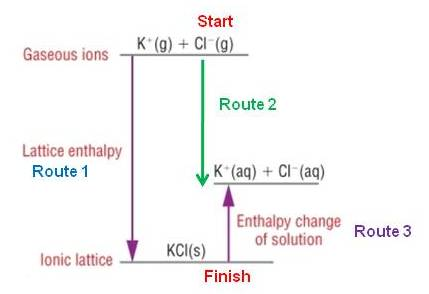Route 1    =     Route 2        +        - Route 3Route 1    =     Route 2        -          Route 3 DHqLE      =      Route 2        -          DHqs Route 2 is made up of 2 steps: DHqLE     =       (SUM OF DHqhyd ions)       -          DHqs DHqLE     =       (- 322     +     - 363)       -          +26 DHqLE     =       (- 322     -      363)       -          26 DHqLE     =       - 685            -          26 DHqLE     =       - 711  Kj mol-1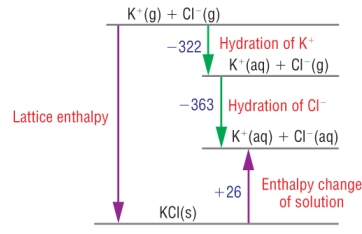Qu 1  P175

Understanding hydration and lattice enthalpies

Lattice enthalpies

• Lattice enthalpy is due to strong electrostatic forces of attraction between oppositely charged ions.

• This means that 2 things can effect the value of the lattice enthalpy:

1.      Ionic size

2.      Ionic charge

Ionic size

• Small ions can pack together closer.

• This gives a stronger electrostatic force of attraction.

• As ions become larger down a group, the attraction decreases.

• This means that the lattice enthalpy will be less exothermic: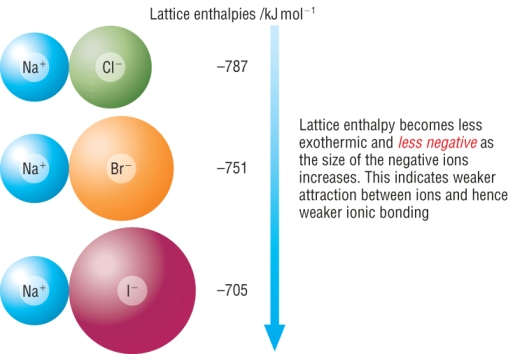Ionic charge

• The larger the charge on the ion, the stronger electrostatic force of attraction between the ions.

• As the ionic charge increases, the electrostatic forces of attraction also increases.

• This means that the lattice enthalpy will be more exothermic:Enthalpy change of hydration:

• Enthalpy change of hydration is due to attractive forces between ions and water.

• This means that 2 things can effect the value of the lattice enthalpy:

1.      Ionic size

2.      Ionic charge

Ionic size

• Small ions attract water molecules closer.

• This gives a stronger force of attraction.

• As ions become larger down a Group, the attraction decreases.

• This means that the hydration enthalpy will be less exothermic:

 Down the group, size increases, DHqhyd gets less exothermic Ion DHqhyd Cl- -363 Br- -336 I- -295

Ionic charge

• A greater charge on ions attract water molecules stronger.

• This gives a stronger force of attraction.

• The ions also become smaller across a Period, the attraction increases further.

• This means that the hydration enthalpy will be more exothermic:

 Across a Period, charge increases and size decreases, DHqhyd gets more exothermic Ion DHqhyd Na+ -406 Mg2+ -1921 Al3+ -4665

Qu 1 - 3  P177

Entropy

What is entropy:

• Entropy is a measure of disorder in a system.

• There is always some degree of disorder as particles always have some sort of motion, so values are always positive.

• As motion is related to enthalpy (energy) then the 2 are related.

• As entropy is due to disorder and so enthalpy (energy) it can be described as the dispersal of energy.

Entropy:

• There are many naturally occurring events that lead to disorder:

Melting ice cream

Smell of cooking spreading

• These are occasions when natural processes lead to disorder.

• There is a tendency in nature for processes to lead to disorder:

Your bedroom

Expanding Universe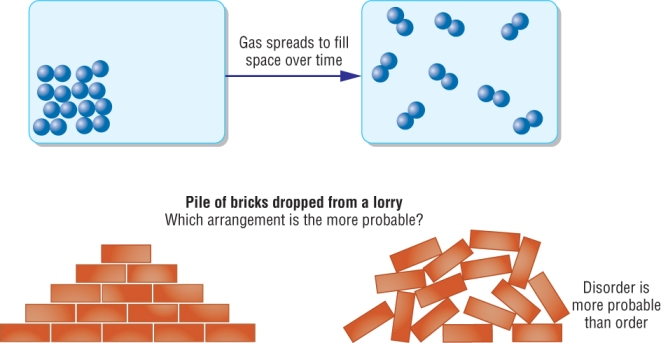What can entropy tell us?

• All these processes start off ordered and become disordered

• The entropy starts of with a low value (ordered) and leads to a higher value (disordered)

• This means that reactants and products will have an entropy content.

• As Chemists we are interested in the change in entropy of a chemical system.

• This gives us an idea as to whether a reaction will occur or not

 Order State Explanation Entropy Ordered (O) Solids Vibrating in fixed position Low Disordered (DO) Liquids/ Aqueous Free moving but closely packed Medium Very Disordered (VDO) Gases As far apart as possible, moving lots High

Using Entropy:

 Reaction NH4NO3(s) + aq à NH4+(aq) + NO3-(aq) Order Ordered Disordered à Disordered Disordered Overall Ordered à Disordered LIKELY TO HAPPEN

 Reaction MgCO3(s) à MgO(s) + CO2(g) Order Ordered à Ordered Very disordered Overall Ordered à Disordered LIKELY TO HAPPEN

Calculating entropy:

• Standard entropy content of a substance can be looked up in a data book.

• They have the units J Mol K-1

• The difference in entropy content between the products and reactants will give a value.

DSqsystem        =         SSqproducts         -           SSqreactants

• If the value is positive it means that the system is moving to more disorder - LIKELY TO HAPPEN

• If the value is negative it means that the system is moving towards more order - UNLIKELY TO HAPPEN

Worked example:

• For the reaction:

 Reaction N2(g) + 3H2(g) à 2NH3(g) Entropy content + 192 + 131 + 193

DSqsystem        =         SSqproducts         -           SSqreactants

DSqsystem        =       (2 x +193)   -       [ (+192)  +  (3 x +131) ]

DSqsystem        =       -199 J K-1 mol-1

Qu 1 - 2  P179

Free energy

Spontaneous process

• Spontaneous reactions occur on their own and move to products with a lower energy and more stability.

Exothermic reactions: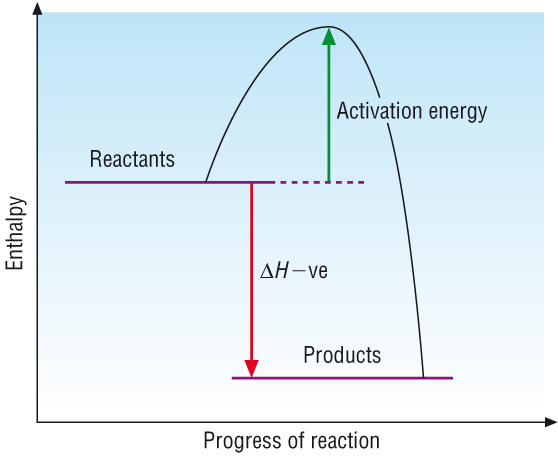Are usually spontaneous at room temperature. The enthalpy content decreases during the reaction and the excess energy being released to the surroundings. There is an increase in stability.

Endothermic reactions: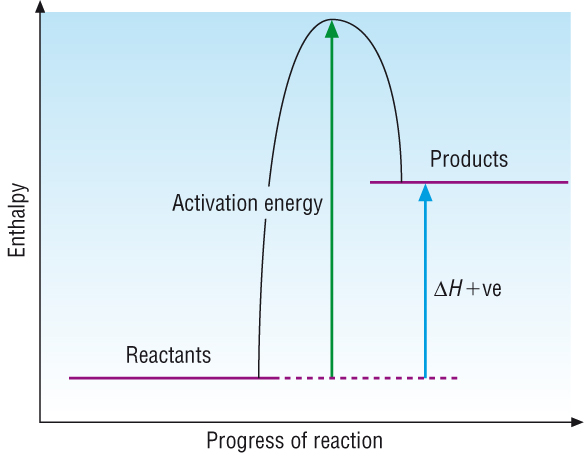Some endothermic reactions are also spontaneous at room temperature. The enthalpy content increases during the reaction with energy being taken in from the surroundings. Overall this must also increase in stability.
• The fact that endothermic reactions are spontaneous too means that there must be more than just enthalpy involved in increasing stability.
• A reaction is spontaneous if a chemical system becomes more stable and its overall energy increases
• In fact stability is derived from the contributions from enthalpy  and entropy.
 Enthalpy Deals with the contribution of energy of the surroundings Entropy Deals with the contribution of energy of the system

Energy from entropy

• The entropy contribution to the overall energy depends on temperature, T in kelvins.

Energy derived from entropy  =  TDS

• As temperature increases, the energy derived from entropy becomes more significant.

Free energy and feasibility:

• 3 factors contribute to whether a reaction will be spontaneous:
 1 Temperature in k T 2 Entropy change of the system DS 3 Enthalpy change with the surroundings DH
• The relationship between these 3 factors is expressed by Gibbs Free energy, DG:
 DG = DH - TDS
 Surroundings System
• For there to be a decrease in (free) energy, leading to more stability:

DG < 0    Negative

 DH DS DG Feasibility / spontaneous (-)ve (+)ve (-)ve Feasible (+)ve (-)ve (+)ve Never feasible (-)ve (-)ve (-)ve at low temps Feasible at low temps (+)ve (+)ve (-)ve at high temps Feasible at high temps
• Most exothermic reactions are feasible / spontaneous as enthalpy contributes more to DG.

How do endothermic reactions take place:

 DG = DH - TDS
• For an endothermic reaction to take place, DS must be positive
• The temperature must be high enough to make, TDS   >   DH
 DG = DH - TDS
 DH < TDS
 (+)ve < TDS
• This will make DG negative and therefore spontaneous.

Example:

At what temperature will ZnCO3 decompose?

 Reaction ZnCO3(s) à ZnO(s) + CO2(g) DH = +71 KJ mol-1 Entropy, DS +82 +44 +214

1)  Calculate DSqsystem:

DSqsystem        =         SSqproducts         -           SSqreactants

DSqsystem        =       (+44  +  +214)   -        +82

DSqsystem        =       176 J K-1 mol-1

DSqsystem        =       0.176 KJ K-1 mol-1  COMMON ERROR - MUST COVERT TO KJ

2)  Assume DG = 0 to calculate T:

 DG = DH - TDS
 0 = + 71 - T  x  0.176
 T  x  0.176 = + 71
 T = + 71
 0.176
 T = 403 k (-273 for oC)
 T = 130 oC

Qu 1 - 2  P181  /  1 - 4  P195  /  1 - 7  P196 - 198